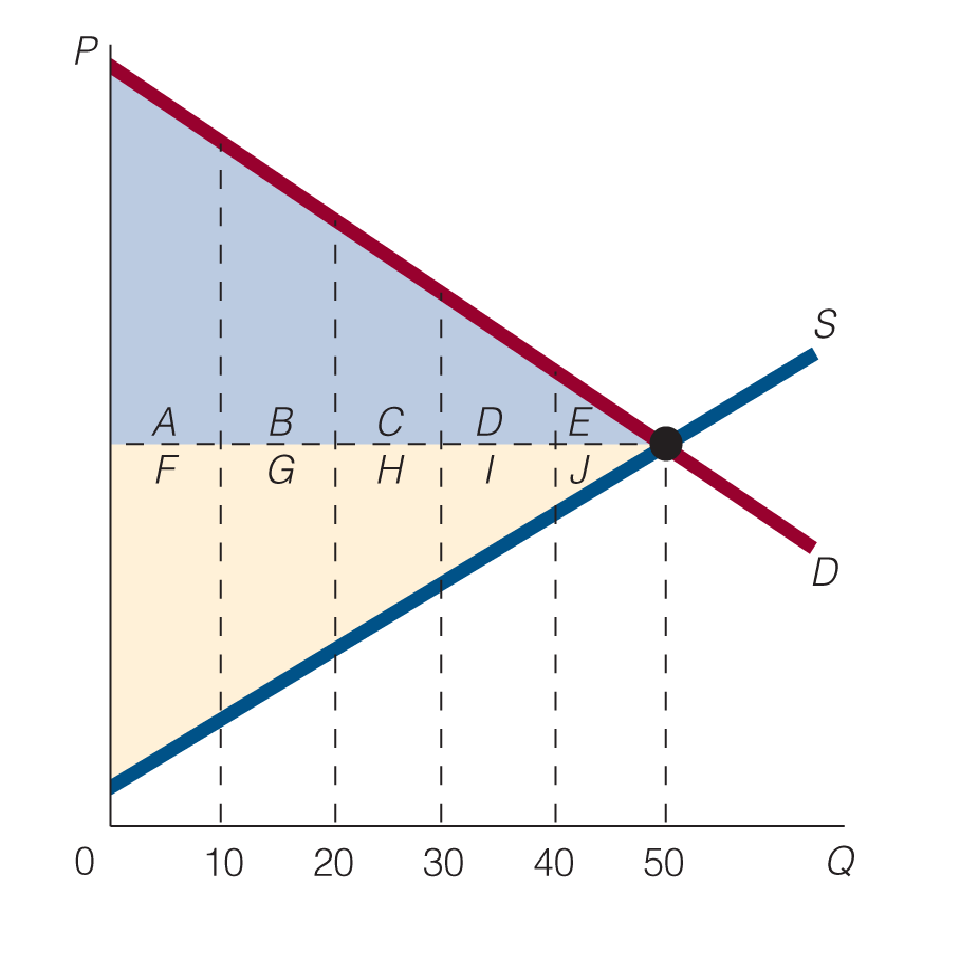Chapter 3, Problem 5WNG

Chapter
Section
Textbook Problem

At equilibrium in the following figure, what area(s) represent consumers’ surplus? producers’ surplus?To determine

Identify the areas of consumer surplus and producer surplus.

Explanation

Figure 1 shows the consumer surplus and producer surplus as follows:

In Figure 1, the vertical axis measures the price and the horizontal axis measures the quantity demanded. The upward sloping curve is the supply curve and the downward sloping curve is the demand curve. The sizes of the consumer surplus and producer surplus are determined by slopes of the supply curve and demand curve...

Still sussing out bartleby?

Check out a sample textbook solution.

See a sample solution

The Solution to Your Study Problems

Bartleby provides explanations to thousands of textbook problems written by our experts, many with advanced degrees!

Get Started

Find more solutions based on key concepts